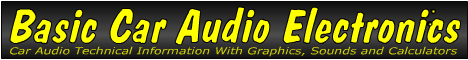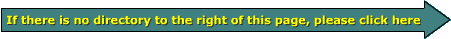Email   Home Pagex

 Web www.bcae1.com www.bcot1.com
Use F11 to go to full screen
Translate.

Numerical Prefixes

Milli is a common prefix used in electronics and car audio. It refers to 1/1000 of something. For example, one millivolt is .001 volt or 1/1000 of one volt. Micro refers to one millionth of something. It is commonly used in stating the value of capacitors. For example, a capacitor with a value of one microfarad has one millionth of the capacitance of a one farad capacitor.

In the following table, I'll use the unit 'watts' to show how the prefixes affect the nomenclature.

 Common Prefixes Used in Electronics 1 terawatt = 1,000,000,000,000 Watts 1 gigawatt = 1,000,000,000 Watts 1 megawatt = 1,000,000 Watts 1 kilowatt = 1,000 Watts 1 milliwatt = 0.001 Watts 1 microwatt = 0.000001 Watts 1 nanowatt = 0.000000001 Watts 1 picowatt = 0.000000000001 Watts 1 femtowatt = 0.000000000000001 Watts

• In the following converter:
• You can enter up to a 5 digit number
• Enter a number on the left and click the prefix of that number (x1 for whole numbers). Then click the prefix that you want to convert it to on the right.
• Start out with 'x1' on the left if you don't fully understand how the conversion works. It will be less confusing.
• If you enter '12' and 'x1' on the left then click micro on the right, it will show you that the whole number 12 is equal to 12,000,000 micro somethings.
• If you click '15' and 'micro' on the left then click 'x1' on the right it will tell you that 15 micro (microfarads for example) is equal to 0.000015 (farads).
• To see how many amps 250 milliamps is equal to, enter 250 on the left, click milli on the left and then 'x1' on the right.

 Data Input: femto pico nano micro milli x1 kilo mega giga tera
 Data Output: femto pico nano micro milli x1 kilo mega giga tera

Dimensional Analysis:
When trying to convert a value with one type of unit (such as 1 cubic foot) to another type of units (such as liters), you can use a tool known as dimensional analysis. It's a pretty simple method that reduces errors when making conversions.

As you alread know, you can multiply or divide anything by 'one' and its value doesn't change. You should also know that you can divide a number by itself (or an equivalent value) and the result is equal to one. A volume of 1ft³ is equal to ~28.3 liters. This means that 1ft³/28.3 liters is equal to '1'. If we know a woofer needs 45 liters but you want to know what that is in ft³, you can use dimensional analysis to make the calculations.

?ft³ =
 45 liters1
*
 1 ft³28.3 liters

In the following fraction you can see how the 'same units' cancel out. This leaves only the values (numbers) and a single type of unit (ft³) to deal with. You then simply multiply straight across to get the final value. To simplify, you'd divide 45 by 28.3. 45 liters is equal to 1.59ft³.

Volume =
 45 liters1
*
 1 ft³28.3 liters

Volume =
 1.59ft³1

 Volume = 1.59ft³

This will work for any number of conversions. If we don't know a direct conversion we can use any number of equivalent values to get to the final value. If we wanted to know how many gallons it would take to fill 1ft³ (and didn't know a direct conversion), we could go the 'long way 'round' to get the answer.

 1 gallon4 quarts
*
 1.057 quarts1 liter
*
 28.3 liters1 ft³

Cancel out like terms (units).

 1 gallon4 quarts
*
 1.057 quarts1 liter
*
 28.3 liters1 ft³

Multiply straight across.

 1 gallon4
*
 1.0571
*
 28.31 ft³

You can see that you'd have 29.91 gallons per 4ft³

 29.91 gallon4 ft³

OR...

 7.48 gallon1 ft³

To see it in a more traditional form, we can multiply both sides by 1ft³/1 (multiplying both sides of the equation by the same number will not change the value of the answer).

 1ft³1
=
 7.48 gallons1

You May Be Interested in My Other Sites
• This site was started for pages/information that didn't fit well on my other sites. It includes topics from backing up computer files to small engine repair to 3D graphics software to basic information on diabetes.
• This site introduces you to macro photography. Macro photography is nothing more than the photography of small objects. It can take quite a while to understand the limitations associated with this type of photography. Without help, people will struggle to get good images. Understanding what's possible and what's not possible makes the task much easier. If you need to photograph relatively small objects (6" in height/width down to a few thousandths of an inch), this site will help.
• If you're interested in air rifles, this site will introduce you to the types of rifles available and many of the things you'll need to know to shoot accurately. It also touches on field target competition. There are links to some of the better sites and forums as well as a collection of interactive demos.
• This site helps anyone new to computers and anyone with a basic understanding of computers with a desire to learn more about the internal components of a computer. If you have a computer that you'd like to upgrade but don't know where to start, this is a good site for you.
• This site is for those who want to begin racing karts but don't fully understand how the various parts work. It's mostly interactive demos that show how the various parts of the kart work.

```

```